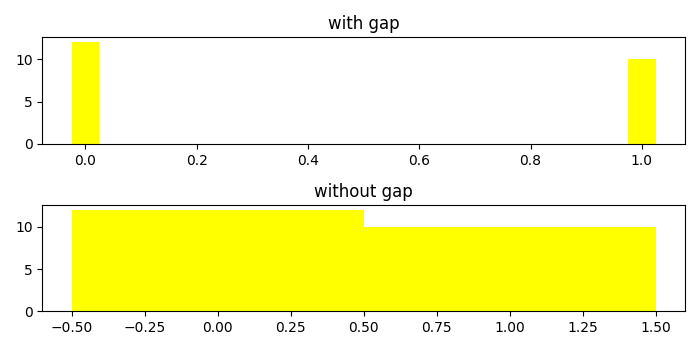# How to remove gaps between bars in Matplotlib bar chart?

To remove gaps between bars, we can change the align value to center in the argument of bar() method.

## Steps

• Create a dictionary called data with two keys, milk and water.

• Get the list of keys and values in the dictionay.

• Using subplots() method, create a figure and add a set of two subplots.

• On axis 2, use bar method to plot bars without gaps. Set the width attribute as 1.0. Set the title using set_title() method.

• Use tight_layout() to adjust the padding between and around the subplots.

• To display the figure, use show() method.

## Example

import matplotlib.pyplot as plt
plt.rcParams["figure.figsize"] = [7.00, 3.50]
plt.rcParams["figure.autolayout"] = True
data = {'milk': 12, 'water': 10}
names = list(data.keys())
values = list(data.values())
fif, (ax1, ax2) = plt.subplots(2)
ax1.bar(range(len(data)), values, align='center', width=.05, color='yellow')
ax1.set_title("with gap")
ax2.bar(range(len(data)), values, align='edge', width=1.0, color='yellow')
ax2.set_title("without gap")
plt.tight_layout()
plt.show()

## Output